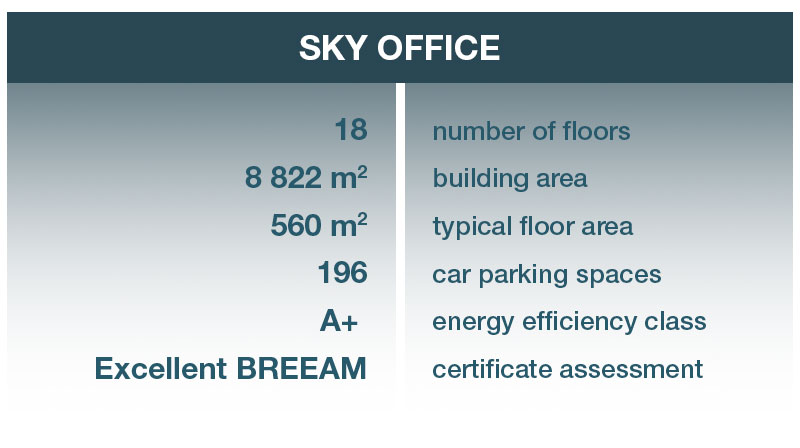S o l a r   p o w e r
p l a n t sB u s i n e s s
c e n t e r   c a f eE l e c t r i c   c a r
c h a r g i n g    s t a t i o n sRoute to